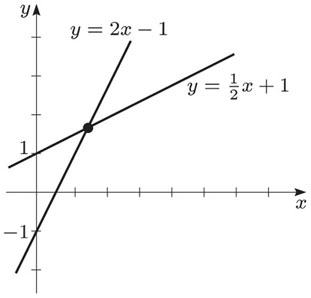# How to Find Point of Intersection of Two Lines

Finding the point of intersection of two lines has many important application such as in Ray-Tracing Simulation.  Two lines always intersect at some point unless they are absolutely parallel, like the rails of a railway track. We start with writing the equations of the two lines in slope-intercept form.

y1=b1+m1*x1

y2=b2+m2*x2Here m1 and m2 are the slopes of the two lines and b1 and b2 are their y-intercepts. At the point of intesection y1=y2, so we have.

b1+m1*x1=b2+m2*x2

But at  the point of intersection x1=x2 as well, so replacing x1 and x2 with x we have.

b1+m1*x=b2+m2*x

or

b1-b2=-x*(m1-m2)

or

x=-(b1-b2)/(m1-m2)

Once the x-component of the point of intersection is found we can easily find the y-component by substituting x in any of the two line equations above.

y=b1+m1*x

In future posts we would like to discuss the cases of intersection of two surfaces and the intersection of two volumes.#### Author: Yasir Ahmed (aka John)

More than 20 years of experience in various organizations in Pakistan, USA and Europe. Worked as Research Assistant within Mobile and Portable Radio Group (MPRG) of Virginia Tech and was one of the first researchers to propose Space Time Block Codes for eight transmit antennas. The collaboration with MPRG continued even after graduating with an MSEE degree and has resulted in 12 research publications and a book on Wireless Communications. Worked for Qualcomm USA as an Engineer with the key role of performance and conformance testing of UMTS modems. Qualcomm is the inventor of CDMA technology and owns patents critical to the 5G and 4G standards.

2.67 avg. rating (60% score) - 3 votes